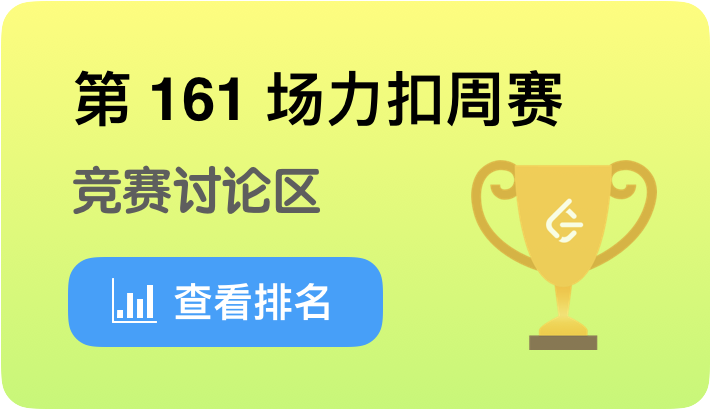🏆 第 161 场力扣周赛4 分 - 交换字符使得字符串相同
4 分 - 统计「优美子数组」
5 分 - 移除无效的括号
6 分 - 检查「好数组」

class Solution:
'''161th week'''
def minRemoveToMakeValid(self, s):
"""
1249. 移除无效的括号
删除最少数目的括号等价于保留最多的括号，即尽可能保留括号，即竟可能匹配更多的有效(左括号在右括号左边)左右括号对。
设置一个未匹配左括号数，
每当出现一个右括号，看未匹配左括号数是否不为0，若不为0即可匹配，未匹配左括号数减1，反之，跳过这个右括号，
每当出现一个左括号，未匹配左括号数加1
"""
self.max_num = -1
self.new_idx = []
self.match_idx = []
k = 0
rest_lbck_num = 0
s_list = []
n = len(s)
while k<n:
if s[k]=='(':
rest_lbck_num+=1
s_list.append(s[k])
elif s[k]==')':
if rest_lbck_num>0:
rest_lbck_num-=1
s_list.append(s[k])
else:
s_list.append(s[k])
k+=1
k = len(s_list)-1
while rest_lbck_num>0:
if s_list[k]=='(':
s_list.pop(k)
rest_lbck_num-=1
k-=1
return "".join(s_list)

def isGoodArray(self, nums):
"""
1250. 检查「好数组」
裴蜀定理:若a,b是整数,且gcd(a,b)=d，那么对于任意的整数x,y,ax+by都一定是d的倍数，特别地，一定存在整数x,y，使ax+by=d成立。
gcd(x,y)=d <=> ax+by=d
gcd(nums[n-1],gcd(nums[n-2],...))=1
"""
def gcd(a,b):
"""
求a和b的最大公约数
a<-b
b<-a%b
"""
if b==0:
return a
else:
return gcd(b,a%b)
n = len(nums)
if n<=0:
return False
d = gcd(nums,nums)
for num in nums[1:]:
d = gcd(d,num)
return d == 1

def numberOfSubarrays(self, nums, k):
"""
1248. 统计「优美子数组」
给你一个整数数组 nums 和一个整数 k。
如果某个 连续 子数组中恰好有 k 个奇数数字，我们就认为这个子数组是「优美子数组」。
请返回这个数组中「优美子数组」的数目。
解题思路：
1.找到第l0个奇数下标a0
2.找到第l1个奇数下标a1
3.找到第lk个奇数下标ak
4.找到第1k+1个奇数下标ak+1
5.包含nums[i1:jk]满足条件的数组数为(a1-a0)*(ak+1-ak)
6.总和为sum((a[i]-a[i-1])*(a[i+k]-a[i+k-1])) i=1,..,m+1-k 第0个奇数为下标为-1的数,第m个奇数为下标为n的数
"""
odd_idx_list = []
n = len(nums)
for i in range(n):
if nums[i]%2==1:
odd_idx_list.append(i)
m = len(odd_idx_list)
if m<k:
return 0
odd_idx_list = [-1]+odd_idx_list+[n]
m+=2
ans = 0
for i in range(1,(m-1-k)+1):
ans+=(odd_idx_list[i]-odd_idx_list[i-1])*(odd_idx_list[i+k]-odd_idx_list[i+k-1])
return ans

def minimumSwap(self, s1, s2):
"""
1247. 交换字符使得字符串相同
有两个长度相同的字符串 s1 和 s2，且它们其中 只含有 字符 "x" 和 "y"，你需要通过「交换字符」的方式使这两个字符串相同。
每次「交换字符」的时候，你都可以在两个字符串中各选一个字符进行交换。
交换只能发生在两个不同的字符串之间，绝对不能发生在同一个字符串内部。也就是说，我们可以交换 s1[i] 和 s2[j]，但不能交换 s1[i] 和 s1[j]。
最后，请你返回使 s1 和 s2 相同的最小交换次数，如果没有方法能够使得这两个字符串相同，则返回 -1 。
0.两字符串长度不等，返回-1
1.两个(x,y)或(y,x)只需要交换一次 (优先交换l1+l2次) l1为(x,y)的对数,l2为(y,x)的对数
2.只剩余(y,x)和(x,y)需要交换两次(剩余交换2次) 返回总交换次数2*(l1+l2)+2
3.2)操作中(y,x)和(x,y)有且只找到一个，则无法使得这两个字符串相同，则返回 -1
"""
if not s1 and not s2:
return 0
if len(s1)!=len(s2):
return -1
l1,l2 = 0,0
for ch1,ch2 in zip(s1,s2):
if (ch1,ch2) == ('x','y'):
l1+=1
elif (ch1,ch2) == ('y','x'):
l2+=1
if l1%2==1 and l2%2==1:
return l1//2+l2//2+2
elif l1%2==1 or l2%2==1:
return -1
else:
return l1//2+l2//2